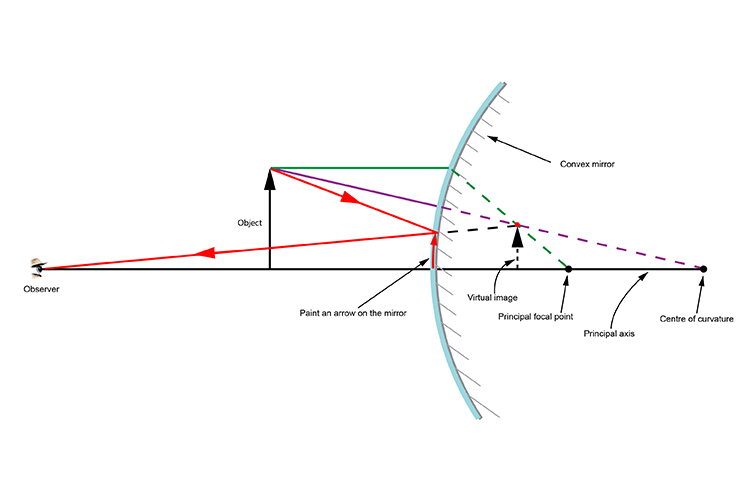# Convex mirror – Ray diagram rules

Below are the ray diagram rules for convex mirrors, which allow you to predict where the light rays from an object will travel and then focus in the eye of an observer.

Rule 1. Take a line from an object parallel to the principal axis and where it touches the convex mirror take another line to the principal focal point.

Rule 2. Take a line from the object and pass it through the centre of curvature of the convex mirror.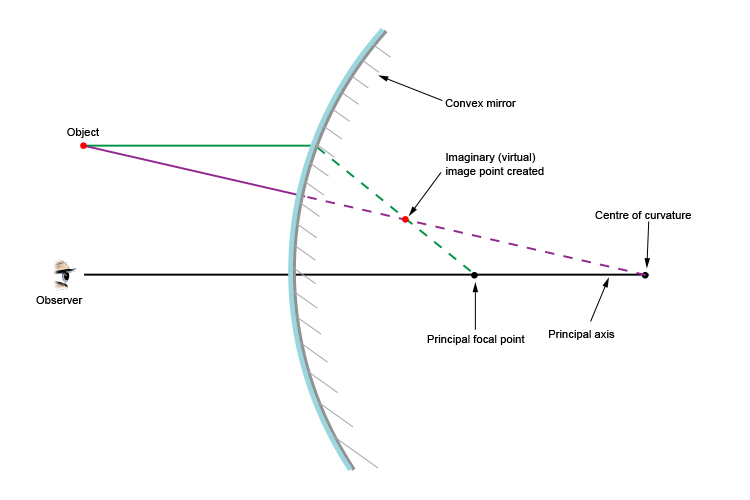NOTE: This only works when the principal axis travels from the eye to the centre.

The point where the two lines cross is where you create an imaginary (a virtual) position. This point can now be used to predict where the real rays will pass from the object to the convex mirror and back to the observer.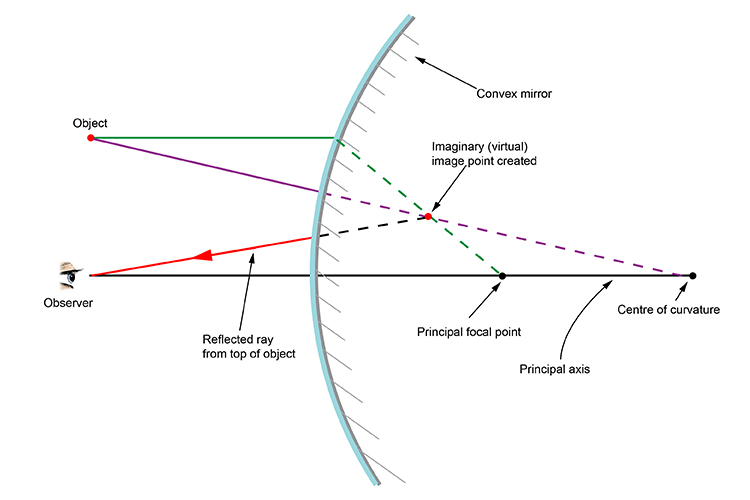Draw a line from the imaginary (virtual) image point that we have created to the observer.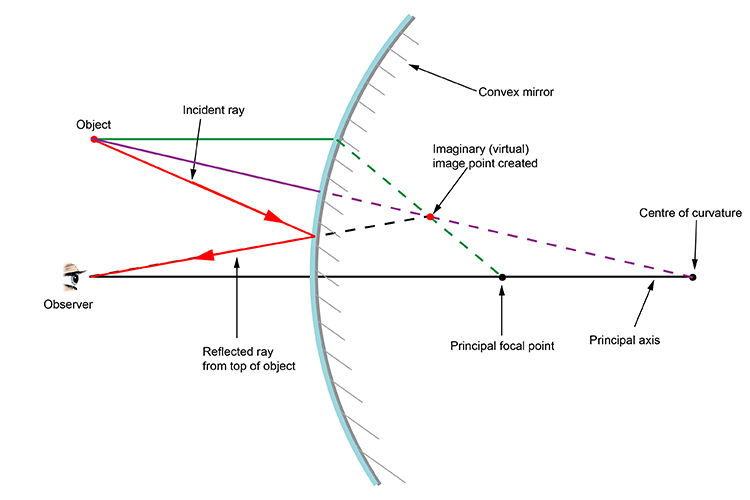Where the line passes the convex mirror, create a new line from the mirror to the object. You will be left with the path that a ray of light will take to the observer.

NOTE: This only works where the principal axis is the line that travels from the observer through the centre of curvature of the convex mirror.

## A new example - Object is an arrow

Lets try another example but this time we will let the object be an arrow but the arrow must be perpendicular (at 90°) to the principal axis.

See below: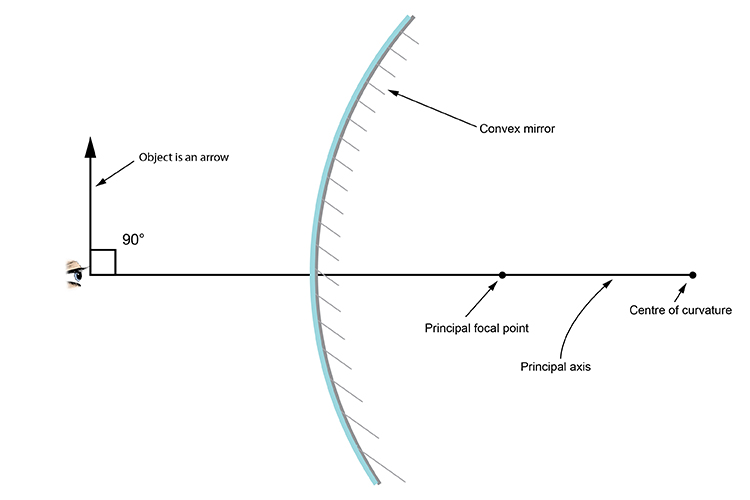Apply Rule 1 and Rule 2 just like before to the tip of the arrow.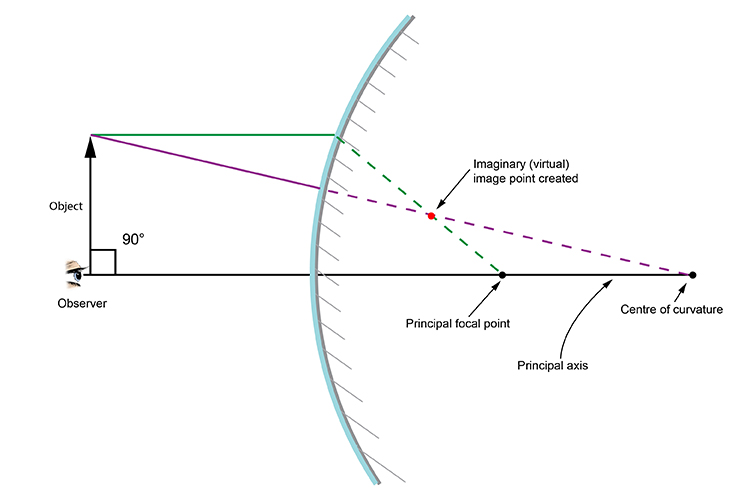Because the object arrow is perpendicular (at right angles) to the principal axis we can drop a line vertically down from the imaginary (virtual) image point created to the principal axis.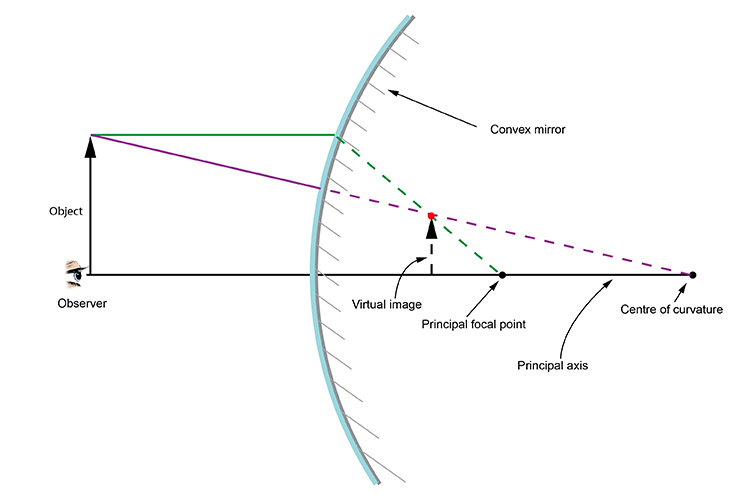This is the classic convex mirror ray diagram that you will see in most physics text books used to create a virtual image.

What most of the text books leave out is that creating this diagram has only one purpose: you can now predict the direction of the incident ray from the object to the observer.

See below: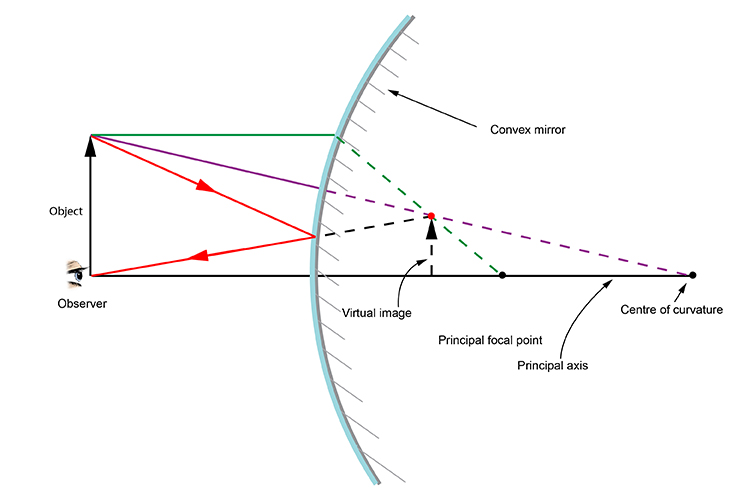If you could ask someone to paint an arrow, in red, where the arrow (object) was on the convex mirror it would look like the diagram below.

If you then removed the object and left the painted arrow, it would look like the original object was still there.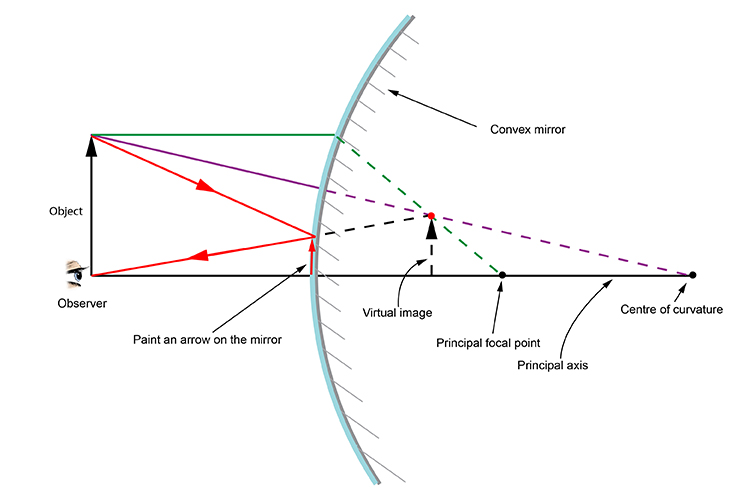You could change the position of the observer anywhere along the principal axis up to the convex mirror and this system still works.

See below: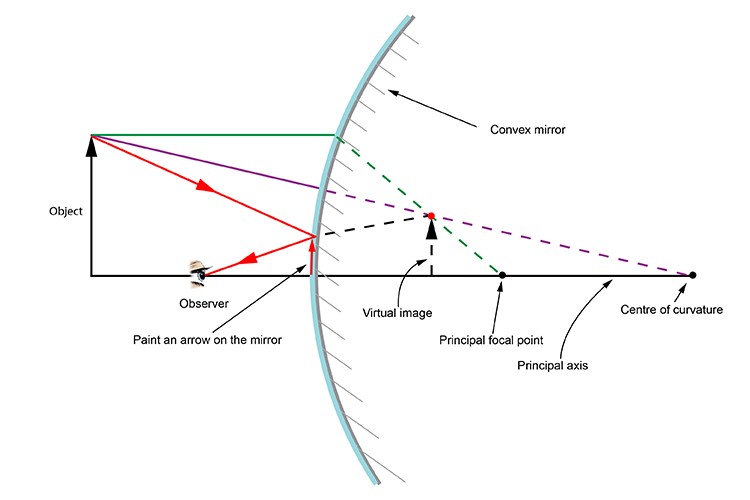It even works when the observer is some distance away from the convex mirror.

See below: